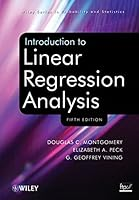# Introduction to Linear Regression Analysis, 5th Edition## Book Description

Praise for the Fourth Edition

"As with previous editions, the authors have produced a leading textbook on regression."
Journal of the American Statistical Association

A comprehensive and up-to-date introduction to the fundamentals of regression analysis

Introduction to Linear Regression Analysis, Fifth Edition continues to present both the conventional and less common uses of linear regression in today’s cutting-edge research. The authors blend both and application to equip readers with an understanding of the basic principles needed to apply regression model-building techniques in various fields of study, including engineering, management, and the sciences.

Following a general introduction to regression , including typical applications, a host of technical tools are outlined such as basic inference procedures, introductory aspects of model adequacy checking, and polynomial regression models and their variations. The book then discusses how transformations and weighted least squares can be used to resolve problems of model inadequacy and also how to deal with influential observations. The Fifth Edition features numerous newly added topics, including:

•  A chapter on regression analysis of time series data that presents the Durbin-Watson test and other techniques for detecting autocorrelation as well as parameter estimation in time series regression models
• Regression models with random effects in addition to a discussion on subsampling and the importance of the mixed model
• Tests on individual regression coefficients and subsets of coefficients
• Examples of current uses of simple linear regression models and the use of multiple regression models for understanding patient satisfaction data.

In addition to Minitab, , and S-PLUS, the authors have incorporated JMP and the freely available R to illustrate the discussed techniques and procedures in this new edition. Numerous exercises have been added throughout, allowing readers to test their understanding of the material.

Introduction to Linear Regression Analysis, Fifth Edition is an excellent book for statistics and engineering courses on regression at the upper-undergraduate and graduate levels. The book also serves as a valuable, robust resource for professionals in the fields of engineering, life and biological sciences, and the social sciences.

Chapter 1. Introduction
Chapter 2. Simple Linear Regression
Chapter 3. Multiple Linear Regression
Chapter 5. Transformations And Weighting To Correct Model Inadequacies
Chapter 6. Diagnostics For Leverage And Influence
Chapter 7. Polynomial Regression Models
Chapter 8. Indicator Variables
Chapter 9. Multicollinearity
Chapter 10. Variable Selection And Model Building
Chapter 11. Validation Of Regression Models
Chapter 12. Introduction To Nonlinear Regression
Chapter 13. Generalized Linear Models
Chapter 14. Regression Analysis Of Time Series Data
Chapter 15. Other Topics In The Use Of Regression Analysis

Appendix A Statistical Tables
Appendix B Data Sets For Exercises
Appendix C Engine Displacement.
Appendix D Introduction To Sas